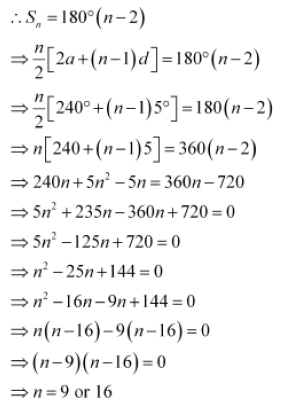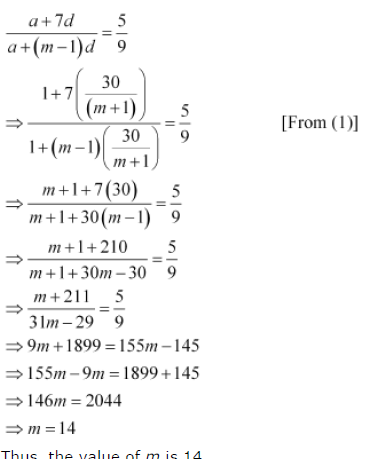## Sunday, 30 June 2013

### The difference between any two consecutive interior angles of a polygon is 5°. If the smallest angle is 120°, find the number of the sides of the polygon.

The difference between any two consecutive interior angles of a polygon is 5°. If the smallest angle is 120°, find the number of the sides of the polygon.

The angles of the polygon will form an A.P. with common difference d as 5° and first term a as 120°.
It is known that the sum of all angles of a polygon with n sides is 180°### A man starts repaying a loan as first installment of Rs. 100. If he increases the installment by Rs 5 every month, what amount he will pay in the 30th installment?

A man starts repaying a loan as first installment of Rs. 100. If he increases the installment by Rs 5 every month, what amount he will pay in the 30th installment?

The first installment of the loan is Rs 100.
The second installment of the loan is Rs 105 and so on.
The amount that the man repays every month forms an A.P.
The A.P. is 100, 105, 110, …
First term, a = 100
Common difference, d = 5
A30 = a + (30 – 1)d
= 100 + (29) (5)
= 100 + 145
= 245
Thus, the amount to be paid in the 30th installment is Rs 245.

### Between 1 and 31, m numbers have been inserted in such a way that the resulting sequence is an A.P. and the ratio of 7th and (m – 1)th numbers is 5:9. Find the value of m.

Between 1 and 31, m numbers have been inserted in such a way that the resulting  sequence is an A.P. and the ratio of 7th and (m – 1)th numbers is 5:9. Find the value of m.

Let A1, A2, … Am be m numbers such that 1, A1, A2, … Am, 31 is an A.P.
Here, a = 1, b = 31, n = m + 2
∴ 31 = 1 + (m + 2 – 1) (d)
⇒ 30 = (m + 1) d
d = 30/(m+1)                ...(1)
A1 = a + d
A2 = a + 2d
A3 = a + 3d …
∴ A7 = a + 7d
Am–1 = a + (m – 1) d
According to the given condition,